### ICSE Class 10 Maths Sample Paper 1# ICSE CLASS 10 MATHS SAMPLE PAPER – 1

ICSE BOARD MATHEMATICS

Sample Paper-1

Time: (Two hours and a half)                                                                  Maximum Marks: 80

● Answers to this Paper must be written on the paper provided separately.

● You will not be allowed to write during the first 15 minutes.

● This time is to be spent in reading the question paper.

● The time given at the head of this Paper is the time allowed for writing the answers.

● Attempt all questions from Section A and any four questions from Section B. All working, including rough work, must be clearly shown and must be done on the same sheet as the rest of the answer.

● Omission of essential working will result in loss of marks.

● The intended marks for questions or parts of questions are given in brackets [ ].

● Mathematical tables are provided.

SECTION A (40 Marks)

Attempt all questions from this Section

Question 1

(a) Find the value of a and b if $x-1 text { and } x-2 text { are factors of } x^{3}-a x+b$                                         

(b) In the figure given below, ABCD is a parallelogram. E is a point on AB. CE intersects the diagonal BD at G and EF is parallel to BC. If AE : EB = 1 : 2 find

(ii) area of triangle BEF : area of triangle ABD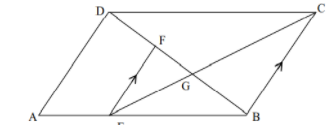(c) On a certain sum of money, the difference between the compound interest for a year, payable half yearly, and the simple interest for a year is Rs 16. Find the sum lent out, if the rate of interest in both cases is 8 %.  

Question 2

(a) Plot the points A(9,6) and B(5,9) on the graph paper. These two points are the vertices of a figure ABCD which is symmetrical about x = 5 and y = 6. Complete the figure on the graph. Write down the geometrical name of the figure.                                                                                                                                                

(b) In the diagram given below $angle EDC$. The tangent drawn to the circle at C makes an angle of 500 with AB produced. Find the measure of $angle ACB$.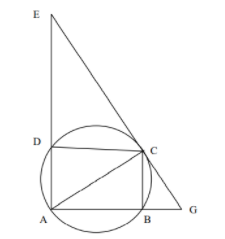(c) PQRS is a square piece of land of side 56 m. Two semi-circular grass covered lawns are made on two of its opposite sides as shown in the figure. Calculate the area of the uncovered portion.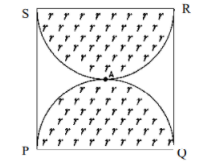Question 3

(a)begin{aligned}&text { If } boldsymbol{A}=left[begin{array}{cc}4 & 4 \-2 & 6end{array}right] text { and } boldsymbol{B}=left[begin{array}{cc}2 & 1 \3 & -2end{array}right] text { find the matrix D such that }\&3 mathrm{~A}-2 mathrm{~B}+2 mathrm{D}=0end{aligned}                        [                                 

(b) A point P(a, b) is reflected in the Y-axis to $mathrm{P}^{1}$ (-3, 1)

i. Write down the values of a and b.

$mathrm{P}^{11}$ is the image of P when reflected in the X axis.

ii. Write down the coordinates of $mathrm{P}^{11}$ .

$mathrm{P}^{111}$ is the image of P when reflected in the line X = 5.

iii. Write down the coordinates of $mathrm{P}^{111}$.                                                                                                

(c) Given :  $boldsymbol{A}={boldsymbol{x}: 3<2 boldsymbol{x}-1<9, boldsymbol{x} in boldsymbol{R}}, boldsymbol{B}={boldsymbol{x}: 11 leq 3 boldsymbol{x}+2 leq 23, boldsymbol{x} in boldsymbol{R}}$  where R is the set of real numbers.

(i) Represent A and B on number lines

(ii) On the number line also mark $boldsymbol{A} cap boldsymbol{B}$.                                                                                                     

Question 4

(a) Without using a trigonometric table calculate:

$4 frac{sin 32^{circ}}{cos 58^{circ}}+5 frac{tan 48^{circ}}{cot 42^{circ}}-8 frac{sec 72^{circ}}{operatorname{cosec} 18^{circ}}$                                                      

(b) Mr. Jacob has a two years recurring deposit account in State Bank of India and deposits Rs.1500 per month. If he receives Rs.37,875 at the time of maturity, find the rate of interest.                                                                                                    

(c) Calculate the arithmetic mean, correct to one decimal place, for the following frequency distribution of marks obtained in a Geometry test.                                                                                                       

 Marks 0-10 10-20 20-30 30-40 40-50 No of students 7 13 15 12 3

SECTION B (40 Marks)

Attempt any four questions from this Section

Question 5

(a) If $left[begin{array}{ll}2 & 4 \6 & 2end{array}right]left[begin{array}{l}3 x \2end{array}right]+2left[begin{array}{l}3 \4end{array}right]=5left[begin{array}{l}4 \yend{array}right]$ find the values of x and y. 

(b) In the diagram given below if AF = 21 cm, CE = 30 cm and FB = 7 cm. Find the volume of the figure.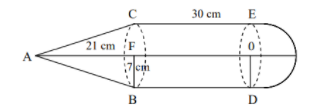(c) A man bought 200 shares each of face value Rs.10 at Rs. 12 per share. At the end of the year, the company from which he bought the shares declares a dividend of 15%. Calculate:

(i) the amount of money invested by the man

(ii) the amount of dividend he received

(iii) the percentage return on his outlay.                                                                                                                                                                                                                                 

Question 6

(a) Solve the following quadratic equation for x and give your answer correct to three significant figures: 

$2 x^{2}-4 x-3=0$

(b) An integer is chosen at random from 1 to 50. Find the probability that the number is:

(i) divisible by 5

(ii) a perfect cube

(iii) a prime numbert                                                                                                                

(c) Find x from the following equation using properties of proportion:                                                        

$frac{x^{2}-x+1}{x^{2}+x+1}=frac{14(x-1)}{13(x+1)}$

Question 7

(a) Bosco wishes to start a 200 $mathrm{m}^{2}$ rectangular vegetable garden. Since he has only 50m barbed wire, he fences three sides of the rectangular garden letting his house compound wall act as the fourth side of the fence. Find the dimensions of the garden.                                                                                                 

(b) Construct a triangle ABC, with AB = 6 cm, BC = 7 cm and ABC = 60 . Locate by construction the point P such that

(i) P is equidistant from B and C.

(ii) P is equidistant from AB and BC

(iii) Measure and record the length of PA. ntral Bank of India.                                                                 

(c) Mr. A. Ramchander has an account with Central Bank of India. The following entries are from his pass book:

 Date Particulars Withdrawal Deposits Balance 05.01.2009 B/F 8000 20.01.2009 To self 2500 04.02.2009 By cash 9000 20.02.2009 By cash 3000 04.03.2009 To self 1000 15.04.2009 By cash 12000

Complete the above page of his passbook and calculate the interest accumulated in four months, January to April at the rate of 3.5% per annum. If the interest is added on 30th April, find his balance on that date. 

Question 8

(a) Prove that $frac{1}{sec x-tan x}+frac{1}{sec x+tan x}=frac{2}{cos x}$                                                                                                                                                                                          

(b) In the figure given below, CD is the diameter of the circle which meets the chord AB at P such that AP = BP = 12 cm. If DP = 8 cm, find the radius of the circle.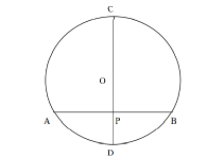(c) Prove that A(2, 1), B(0,3) and C(-2,1) are the three vertices of an isosceles right angled triangle. Hence find the coordinates of a point D, if ABCD is a square.                                                                                                  

Question 9

(a) A fair dice is rolled. Find the probability of getting

(i) 3 on the face of the dice

(ii) an odd number on the face of the dice

(iii) a number greater than 1 on the face of the dice. BC.                                                                          

(b) A (4,2), B(6,8) and C (8,4) are the vertices of a triangle ABC. Write down the equation of the median of the triangle through A.                                                                                                                                                    

(c) The angle of elevation of an aeroplane from a point P on the ground is $60^{circ}$. After 12 seconds from the same point P, the angle of elevation of the same plane changes to $30^{circ}$. If the plane is flying horizontally at a speed of $600 sqrt{3} mathrm{~km} / mathrm{h}$, find the height at which the plane is flying.                                                              

Question 10

 Height (cm) 140-145 145-150 150-155 155-160 160-165 165-170 170-175 No of students 8 12 18 22 26 10 4

(a) The following table shows the distribution of the heights of a group of students:

Use a graph sheet to draw an Ogive for the distribution. Use the Ogive to find:

(i) the inter quartile range

(ii) the number of students whose height is more than 168 cm

(iii) the number of students whose height is less than 148 cm.                                                               

(b) The manufacturer sold a TV to a wholesaler for Rs.7000. The wholesaler sold it to a trader at a profit of Rs.1000. If the trader sold it to the customer at a profit of Rs.1500, find:

(i) the total VAT (value added tax) collected by the state government at the rate of 5%.

(ii) the amount that the customer pays for the TV.                                                                                       

Question 11

(a) In the diagram given below, equation of AB is $x-sqrt{3} y+1=0$ and equation of AC is x – y – 2 = 0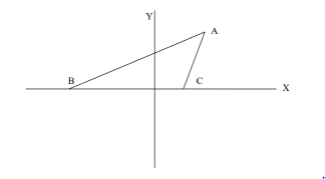(i) Write down the angles that the lines AC and AB make with the positive direction of X- axis.

(ii) Find $angle B A C text { . }$

(b) In the figure given below, O is the center of the circle. Chord CD is parallel to the diameter AB. If $angle mathrm{ABC}=35, text { calculate } angle mathrm{CED}$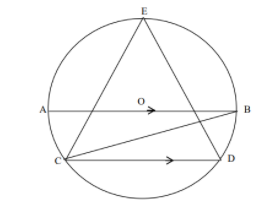(c) Construct a triangle ABC, given that AB = 6 cm, BC = 8 cm and median AD = 5 cm. Construct an

incircle to triangle ABC and measure its radius.                                                                                                       

Privacy Settings